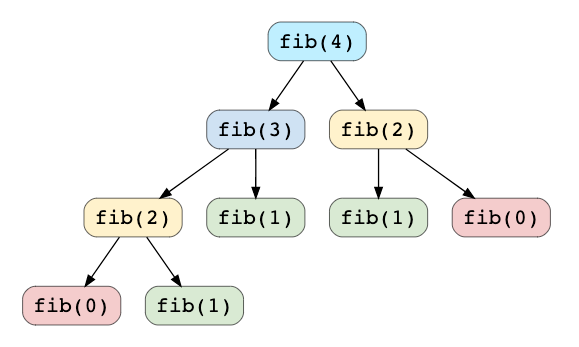# What is Dynamic Programming?

Dynamic Programming (DP) is an algorithmic technique for solving an optimization problem by breaking it down into simpler subproblems and utilizing the fact that the optimal solution to the overall problem depends upon the optimal solution to its subproblems.

Let’s take the example of the Fibonacci numbers. As we all know, Fibonacci numbers are a series of numbers in which each number is the sum of the two preceding numbers. The first few Fibonacci

numbers are 0, 1, 1, 2, 3, 5, and 8, and they continue on from there.

If we are asked to calculate the nth Fibonacci number, we can do that with the following equation,

Fib(n) = Fib(n-1) + Fib(n-2), for n > 1

As we can clearly see here, to solve the overall problem (i.e. Fib(n)), we broke it down into two smaller subproblems (which are Fib(n-1) and Fib(n-2)). This shows that we can use DP to solve this problem.

## Characteristics of Dynamic Programming

Before moving on to understand different methods of solving a DP problem, let’s first take a look at what are the characteristics of a problem that tells us that we can apply DP to solve it.

1. Overlapping Subproblems

Subproblems are smaller versions of the original problem. Any problem has overlapping sub-problems if finding its solution involves solving the same subproblem multiple times. Take the example of the Fibonacci numbers; to find the fib(4), we need to break it down into the following sub-problems:Recursion tree for calculating Fibonacci numbers

We can clearly see the overlapping subproblem pattern here, as fib(2) has been called twice and fib(1) has been called three times.

2. Optimal Substructure Property

Any problem has optimal substructure property if its overall optimal solution can be constructed from the optimal solutions of its subproblems. For Fibonacci numbers, as we know,

Fib(n) = Fib(n-1) + Fib(n-2)

This clearly shows that a problem of size ‘n’ has been reduced to subproblems of size ‘n-1’ and ‘n-2’. Therefore, Fibonacci numbers have optimal substructure property.

## Dynamic Programming Methods

DP offers two methods to solve a problem:

1. Top-down with Memoization

In this approach, we try to solve the bigger problem by recursively finding the solution to smaller sub-problems. Whenever we solve a sub-problem, we cache its result so that we don’t end up solving it repeatedly if it’s called multiple times. Instead, we can just return the saved result. This technique of storing the results of already solved subproblems is called Memoization.

We’ll see this technique in our example of Fibonacci numbers. First, let’s see the non-DP recursive solution for finding the nth Fibonacci number:

Java:

Python:

As we saw above, this problem shows the overlapping subproblems pattern, so let’s make use of memoization here. We can use an array to store the already solved subproblems:

Java:

Python:

2. Bottom-up with Tabulation

Tabulation is the opposite of the top-down approach and avoids recursion. In this approach, we solve the problem “bottom-up” (i.e. by solving all the related sub-problems first). This is typically done by filling up an n-dimensional table. Based on the results in the table, the solution to the top/original problem is then computed.

Tabulation is the opposite of Memoization, as in Memoization we solve the problem and maintain a map of already solved sub-problems. In other words, in memoization, we do it top-down in the sense that we solve the top problem first (which typically recurses down to solve the sub-problems).

Let’s apply Tabulation to our example of Fibonacci numbers. Since we know that every Fibonacci number is the sum of the two preceding numbers, we can use this fact to populate our table.

Here is the code for our bottom-up dynamic programming approach:

Java:

Python:

Take a look at Grokking Dynamic Programming Patterns for Coding Interviews for some good examples of DP question and their answers.

We can be reached at Design Gurus.